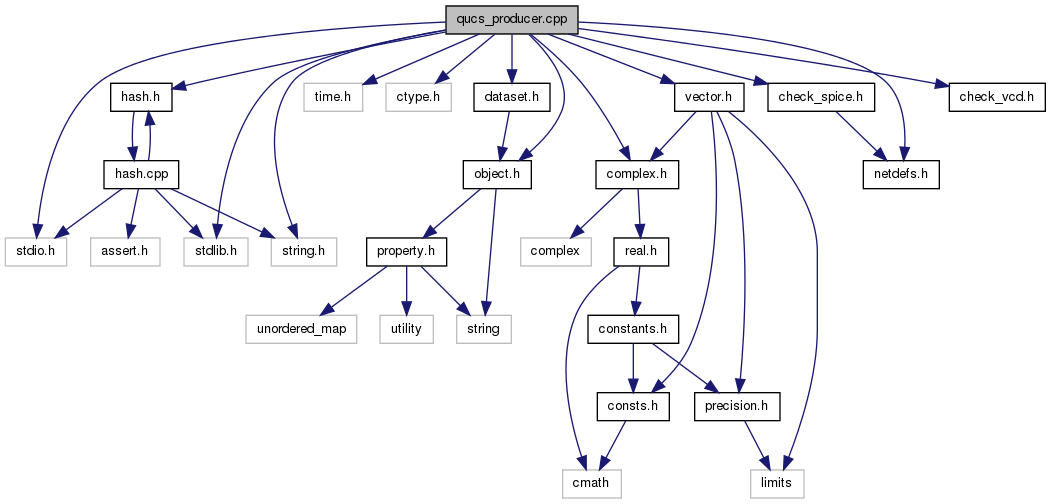Qucs-core  0.0.19
qucs_producer.cpp File Reference
`#include <stdio.h>`
`#include <stdlib.h>`
`#include <time.h>`
`#include <string.h>`
`#include <ctype.h>`
`#include "object.h"`
`#include "complex.h"`
`#include "vector.h"`
`#include "dataset.h"`
`#include "netdefs.h"`
`#include "check_spice.h"`
`#include "check_vcd.h"`
`#include "hash.h"`
Include dependency graph for qucs_producer.cpp:Go to the source code of this file.

struct  device_t

## Functions

int qucs_find_node (struct node_t *root, char *node)
void qucs_add_nodes (struct node_t *node)
void qucs_free_nodes (struct node_t *node)
static void qucs_delete_nodes (void)
static void qucs_collect_nodes (struct definition_t *root)
static void netlist_list_value (struct value_t *value)
static char * netlist_instance (const char *instance)
static void netlist_list_def (struct definition_t *def, const char *prefix)
static void netlist_lister (struct definition_t *root, const char *prefix)
static void netlist_fix_reference (struct definition_t *root)
static void netlist_reference_ground (void)
static struct definition_tnetlist_get_single_subcircuit (struct definition_t *root)
static void netlist_list (void)
void qucs_producer (void)
static struct device_tqucslib_find_device (char *type)
static struct pair_tqucslib_find_prop (struct definition_t *def, const char *key)
static void qucslib_list_device (struct definition_t *def)
void qucslib_producer (void)
void qucsdata_producer_vcd (void)
void qucsdata_producer (dataset *data)

## Variables

FILE * qucs_out = NULL
int qucs_actions = 1
const char * qucs_gnd = "gnd"
qucs::hash< struct node_tqucs_nodes
struct device_t qucs_devices []

## Function Documentation

 static void netlist_fix_reference ( struct definition_t * root ) ` [static]`

Definition at line 165 of file qucs_producer.cpp.

 static struct definition_t* netlist_get_single_subcircuit ( struct definition_t * root ) ` [static, read]`

Definition at line 203 of file qucs_producer.cpp.

 static char* netlist_instance ( const char * instance ) ` [static]`

Definition at line 116 of file qucs_producer.cpp.

 static void netlist_list ( void ) ` [static]`

Definition at line 218 of file qucs_producer.cpp.

 static void netlist_list_def ( struct definition_t * def, const char * prefix ) ` [static]`

Definition at line 123 of file qucs_producer.cpp.

 static void netlist_list_value ( struct value_t * value ) ` [static]`

Definition at line 95 of file qucs_producer.cpp.

 static void netlist_lister ( struct definition_t * root, const char * prefix ) ` [static]`

Definition at line 149 of file qucs_producer.cpp.

 static void netlist_reference_ground ( void ) ` [static]`

Definition at line 188 of file qucs_producer.cpp.

 void qucs_add_nodes ( struct node_t * node )

Definition at line 63 of file qucs_producer.cpp.

 static void qucs_collect_nodes ( struct definition_t * root ) ` [static]`

Definition at line 87 of file qucs_producer.cpp.

 static void qucs_delete_nodes ( void ) ` [static]`

Definition at line 82 of file qucs_producer.cpp.

 int qucs_find_node ( struct node_t * root, char * node )

Definition at line 54 of file qucs_producer.cpp.

 void qucs_free_nodes ( struct node_t * node )

Definition at line 72 of file qucs_producer.cpp.

 void qucs_producer ( void )

Definition at line 259 of file qucs_producer.cpp.

 void qucsdata_producer ( dataset * data )

Definition at line 553 of file qucs_producer.cpp.

 void qucsdata_producer_vcd ( void )

Definition at line 530 of file qucs_producer.cpp.

 static struct device_t* qucslib_find_device ( char * type ) ` [static, read]`

Definition at line 470 of file qucs_producer.cpp.

 static struct pair_t* qucslib_find_prop ( struct definition_t * def, const char * key ) ` [static, read]`

Definition at line 483 of file qucs_producer.cpp.

 static void qucslib_list_device ( struct definition_t * def ) ` [static]`

Definition at line 494 of file qucs_producer.cpp.

 void qucslib_producer ( void )

Definition at line 521 of file qucs_producer.cpp.

## Variable Documentation

 int qucs_actions = 1

Definition at line 46 of file qucs_producer.cpp.

 struct device_t qucs_devices[]
 const char* qucs_gnd = "gnd"

Definition at line 47 of file qucs_producer.cpp.

 qucs::hash qucs_nodes

Definition at line 49 of file qucs_producer.cpp.

 FILE* qucs_out = NULL

Definition at line 45 of file qucs_producer.cpp.